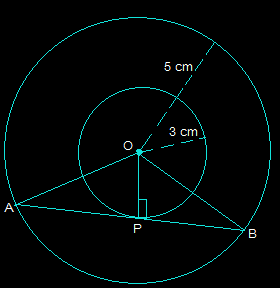Guru

# Two concentric circles are of radii 5 cm and 3 cm. Find the length of the chord of the larger circle which touches the smaller circle. Q.7

• 0

What is easy way to solve the question of class 10th of circles chapter of exercise 10.2 of question no.7, this is an important question for class 10th board exams, please suggest me the best way to solve this question Two concentric circles are of radii 5 cm and 3 cm. Find the length of the chord of the larger circle which touches the smaller circle.

Share

1. Draw two concentric circles with the center O. Now, draw a chord AB in the larger circle which touches the smaller circle at a point P as shown in the figure below.From the above diagram, AB is tangent to the smaller circle to point P.

∴ OP ⊥ AB

Using Pythagoras theorem in triangle OPA,

OA2= AP2+OP2

52 = AP2+32

AP2 = 25-9

AP = 4

Now, as OP ⊥ AB,

Since the perpendicular from the center of the circle bisects the chord, AP will be equal to PB

So, AB = 2AP = 2×4 = 8 cm

So, the length of the chord of the larger circle is 8 cm.

• 0